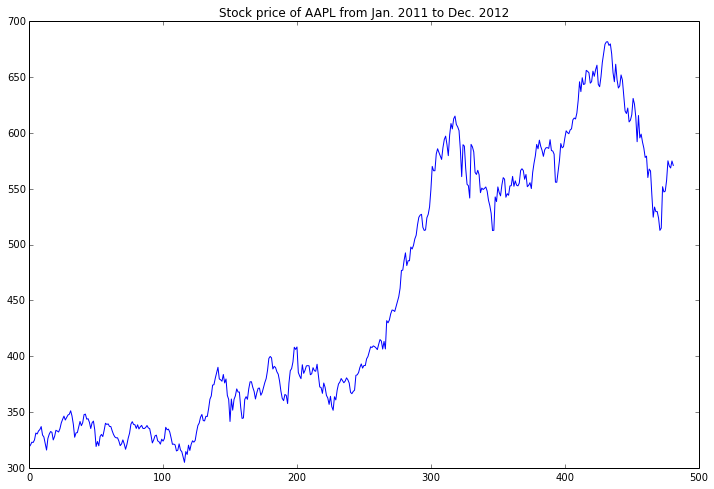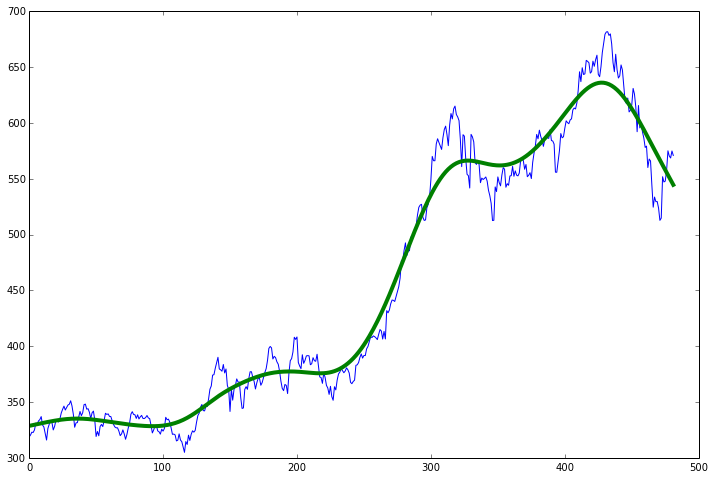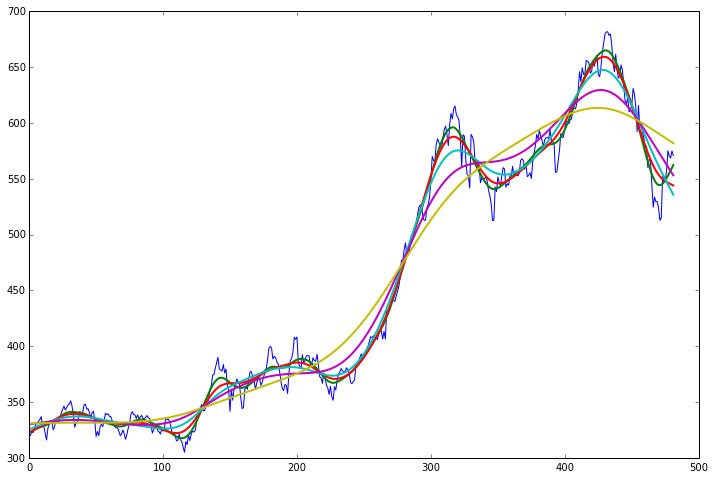-->

## Friday, November 15, 2013

### An Introduction to Trend Filtering 1D Data with the Hodrick-Prescott Filter

Filtering time-series data can be a tricky business. Many times what seems to obvious to our eyes is difficult to mathematically put into practice. A recent technique I've been reading about is called "total variance minimization" filtering or another name would be "$\ell_1$ trend filtering." Another member of this family is the Hodrick-Prescott (HP) trend filter. In all three of these types of filters, we're presented with a set of noisy data, $y = x + z$ (where $x$ is the real signal and $z$ is some noise) and we're trying to fit a more simple model or set of lines to this data. For the TV filter, the type of lines we're fitting to the data are lines that don't have many large "jumps", or mathematically, $\vert (x_{n-1} - x_{n}) \vert$, the difference between two of the fitted values, is small. In HP and $\ell_1$ trend filtering, the goal is to make sure that the 2nd derivative is small. Mathematically this looks like minimizing the term

$$(x_{n-2} - x_{n-1}) - (x_{n-1} - x_{n}) .$$

This makes sense, because the only time that the term above goes to zero is if the data is in a straight line - no "kinks" in the trendline. In HP filtering this term is squared and in $\ell_1$ filtering this term is just the absolute value (the $l_1$ norm). HP filtering fits a kind of spline to the data, while the $\ell_1$ filtering fits a piecewise linear function (straight lines that join at the knots).

The actual cost function looks a bit like this:

$$c(x) = \frac{1}{2}\sum_{i=1}^{n-1} (y_i - x_i)^2 + \lambda P(x)$$

where $P(x)$ is one of the penalty terms we talked about above and $\lambda$ controls the strength of the denoising. Again, remember that $y$ is our observed signal and $x$ is our projected or estimated signal - the trend. Setting $\lambda$ to infinity will cause the fitted line to be a straight line through the data, and setting $\lambda$ to zero will fit a line that looks identical to the original data. In HP filtering the whole function we're minimizing looks like this

$$c(x) = \frac{1}{2}\sum_{i=1}^{n-1} (y_i - x_i)^2 + \lambda\sum_{i = 1}^{n-1} ((x_{n-2} - x_{n-1}) - (x_{n-1} - x_{n}))^2$$

and so $\hat{x} = \arg \min_x c(x)$.

In order to find the minimum value of $\hat{x}$ we can take the derivative of $c(x)$ with respect to $x$ and solve for zero. This isn't too difficult, and having both the terms in the HP filter squared makes this a bit easier - $\ell_1$ filtering is trickier.

Before we compute the derivative, let's rewrite the ending penalty term as a matrix multiplication. In order to compute $((x_{n-2} - x_{n-1}) - (x_{n-1} - x_{n}))^2$ for each value of $n$, we can multiply our array of $x$ values, $x = (x_1, x_2, ..., x_n)^T \in \mathbb R^{n x 1}$, by a matrix that takes the difference. It will look like this

$$D = \begin{bmatrix} \\ 1 & -2 & 1 & & & & \\ & 1 & -2 & 1 & & & \\ & & \ddots & \ddots & \ddots & & \\ & & & 1 & -2 & 1 & \\ & & & & 1 & -2 & 1 \\ \end{bmatrix}$$

So now, $D \in \mathbb R^{N-2 \times N}$ and $Dx$ will now be a $\mathbb R^{N-2 \times 1}$ matrix descring the difference penalty. The cost function can now be rewritten as

$$c(x) = \frac{1}{2}\Vert y - x \Vert^2 + \lambda\Vert Dx\Vert^2$$

Notice that both $\Vert y - x \Vert^2$ and $\Vert Dx \Vert^2$ are single values, since both of the inner quantaties are vectors ($N \times 1$ and $N-2 \times 1$) and so taking the $\ell_2$ norm sums the square of the elements, yielding one value.

If we take the derivative of $c(x)$ w.r.t $x$, we have

$$\frac{\partial c(x)}{\partial x} = -y + x + 2\lambda D^TDx = 0$$

The first part is taking the derivative of $(y - x)(y - x)^T$ (which is a different way of writing the $\ell_2$ norm), and the latter part comes out of the tedious computation of the derivative of the $\ell_2$ norm of $Dx$.

Rearranging this equation yields

$$(x - 2\lambda D^TDx) = y$$

Solving for $x$ gives

$$(I - 2\lambda D^TD)^{-1}y = \hat x$$

This is super handy because it gives us an analytical way of solving for $\hat x$ by just multiplying our $y$ vector by a precomputed transformation matrix - $(I - 2\lambda D^TD)$ is a fixed matrix of size $N \times N$.

An Example

Let's take the stock price of Apple over time. We can import this via the matplotlib (!) module:

In :
from matplotlib import finance
%pylab inline
pylab.rcParams['figure.figsize'] = 12, 8  # that's default image size for this interactive session
import statsmodels.api as sm

Populating the interactive namespace from numpy and matplotlib


In :
d1 = datetime.datetime(2011, 01, 01)
d2 = datetime.datetime(2012, 12, 01)
sp = finance.quotes_historical_yahoo('AAPL',d1,d2,asobject=None)

In :
plot(sp[:,2])
title('Stock price of AAPL from Jan. 2011 to Dec. 2012')

Out:
<matplotlib.text.Text at 0x1106d438>Now that we have the historic data we want to fit a trend line to it. The Statsmodels package has this filter already built in. It comes installed with an Anaconda installation. You can give the function a series of data and the $\lambda$ parameter and it will return the fitted line.

In :
xhat = sm.tsa.filters.hpfilter(sp[:,2], lamb=100000)
plot(sp[:,2])
hold(True)
plot(xhat,linewidth=4.)

Out:
[<matplotlib.lines.Line2D at 0x1539ba90>]The green line nicely flows through our data. What happens when we adjust the regularization? Since we don't really know what value to pick for $\lambda$ we'll try a range of values and plot them.

In :
lambdas = numpy.logspace(3,6,5)  # Generate a logarithmicly spaced set of lambdas from 1,000 to 1 Million
xhat = []
for i in range(lambdas.size):
xhat.append(sm.tsa.filters.hpfilter(sp[:,2],lambdas[i]))  # Return the 2nd argument and apped

In :
plot(sp[:,2])
hold(True)
plot(transpose(xhat),linewidth=2.)

Out:
[<matplotlib.lines.Line2D at 0x1580f908>,
<matplotlib.lines.Line2D at 0x1580fef0>,
<matplotlib.lines.Line2D at 0x158110b8>,
<matplotlib.lines.Line2D at 0x15811240>,
<matplotlib.lines.Line2D at 0x158113c8>]You can see we move through a continuum of highly fitted to loosly fitted data. Pretty nifty, huh? You'll also notice that the trend line doesn't have any sharp transitions. This is due to the squared penalty term. In $\ell_1$ trend filtering you'll end up with sharp transitions. Maybe this is good, maybe not. For filtering data where there's no guarantee that the data will be semi-continuous (maybe some sensor reading), perhaps the TV or $\ell_1$ filter is better. The difficulty is that by adding the $\ell_1$ penalty the function becomes non-differentialable and makes the solution a little more difficult - generally an iterative process.